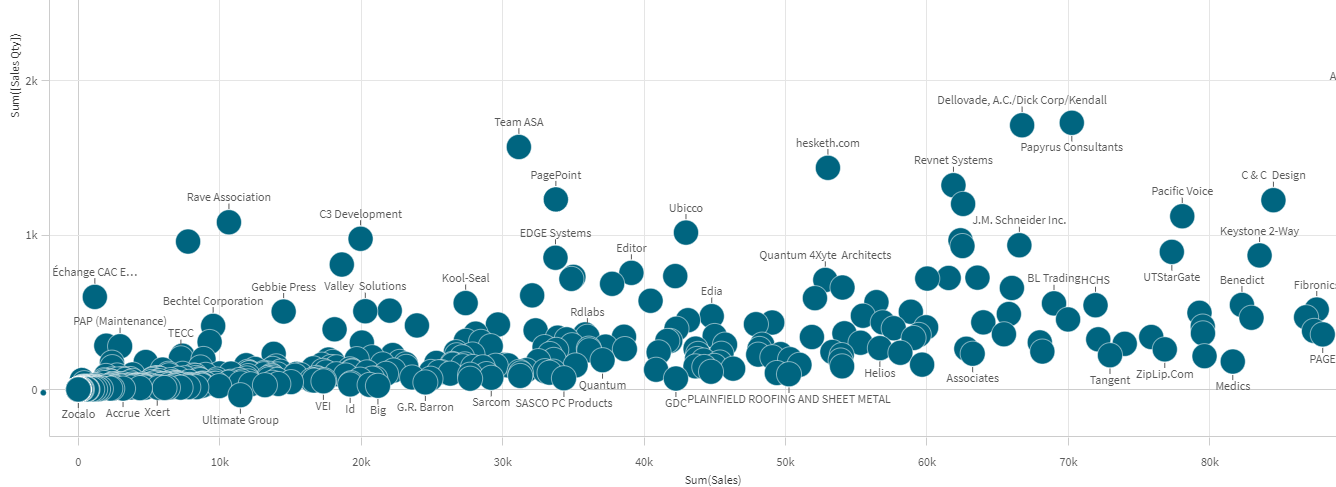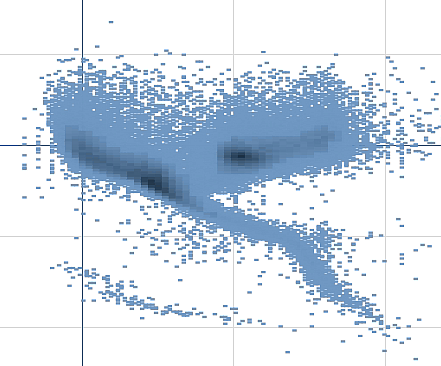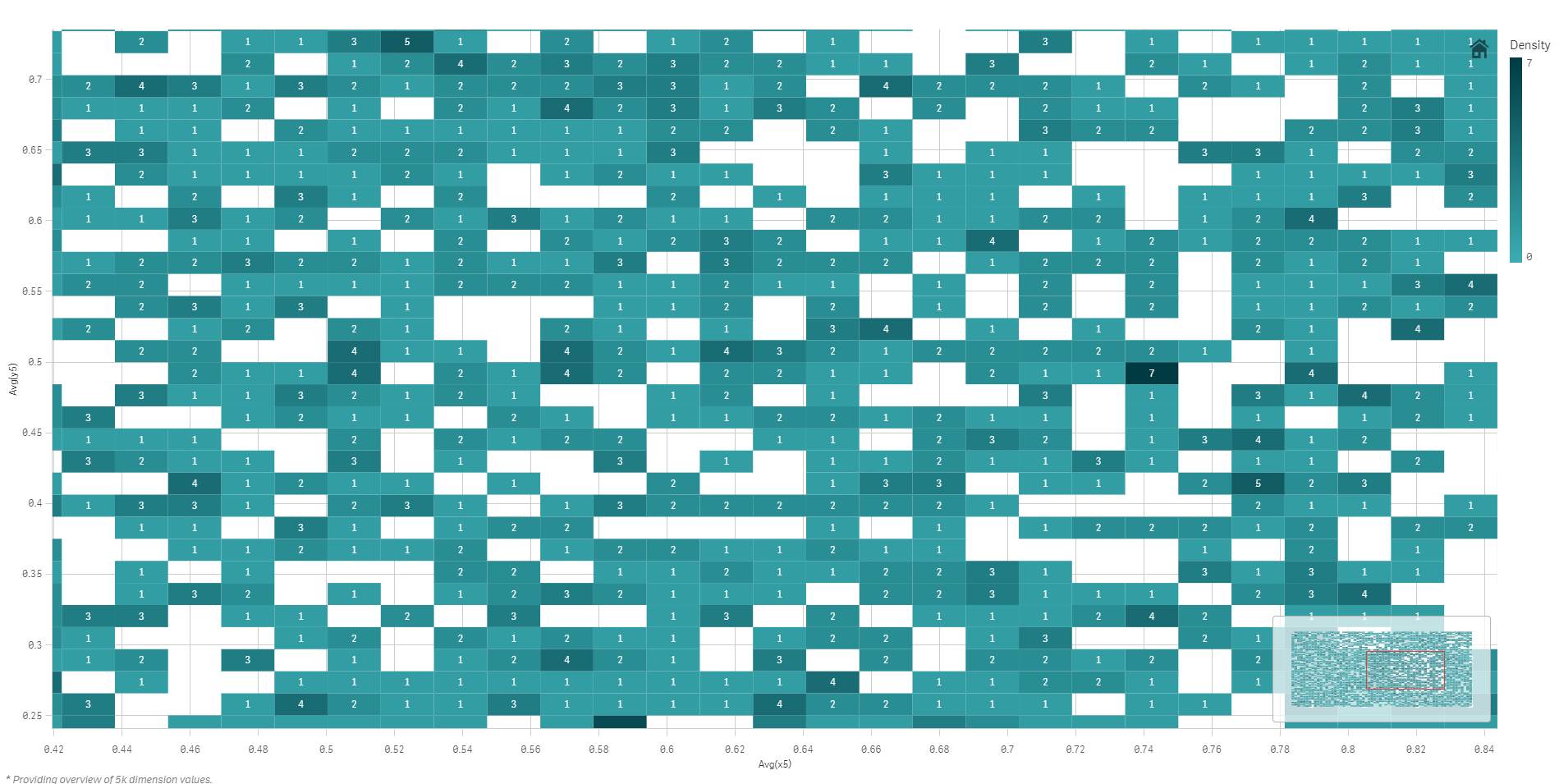# Scatter plot

The scatter plot presents pairs of values from two or three measures.

This is useful when you want to show data where each instance has two numbers, for example, the relationship between Sales and Quantity per Customer. In the scatter plot below, a third measure (Cost) is used to generate the bubble size.

Scatter plot displaying the relationship between Sales and Quantity per Customer.The scatter plot presents values from different measures over one dimension as a collection of points. In most charts, you find your dimension on one of the axes, but for a scatter plot, the dimension is represented by the points in the chart, and the measures are found on each of the two axes. When a third, optional, measure is used, its value is reflected in the bubble size. If you are analyzing large data sets and view compressed data, the density of the data points is reflected by color.

## When to use it

The scatter plot helps you find potential relationships between values, and to find outliers in data sets. The scatter plot is useful when you want to show data where each instance has at least two metrics, for example, average life expectancy and average gross domestic product per capita in different countries.

The scatter plot is a great way to visualize the correlation of two or more measures at the same time. The third measure is an efficient way of differentiating between values and simplifying the identification of, for example, large countries, large customers, large quantities, and so on.

The scatter plot may be difficult to understand for an inexperienced user, because it has measure value on both axes, and the third, optional, measure adds complexity to the interpretation. Make sure a novice can interpret the scatter plot correctly. Using descriptive labels is a good way to make the visualization easier to interpret.

Values may be placed on top of each other and are then not visible until you zoom in.

## Creating a scatter plot

You can create a scatter plot on the sheet you are editing.

In a scatter plot you need one dimension and at least two measures. You can have maximum one dimension and three measures, where the third measure is visualized as bubble size.

1. From the assets panel, drag an empty scatter plot to the sheet.
2. Click Add dimension and select a dimension or a field.
3. Click Add measure and select a measure or create a measure from a field.
4. Click Add measure and select a measure or create a measure from a field.
5. Optionally, if you want bubble size to be set according to a third measure:

Click Add measure and select a measure or create a measure from a field.

When you have created the scatter plot, you may want to adjust its appearance and other settings in the properties panel.

## Display limitations

### Large data sets in scatter plots

If you have a scatter plot with large amounts of data (more than 1000 data points) Qlik Sense uses an algorithm to create an overview of the data, as shown in the scatter plot below. However, if you zoom or make selections so that the number of displayed data points is reduced to less than 1000 data points, the data will be shown as individual bubbles. This switch between compressed view and bubble view is done automatically. The density of the data points is reflected by color.### Zooming and panning

In a scatter plot, you can zoom and pan around in your data. The interaction differs depending on what device you are using. If you are zooming in you can see where in the data set you are located by looking at the mini chart in the bottom right corner. If you zoom in on large data sets you will be able to see the data shown as boxes with values inside. The values represent the number of points in each box. If you zoom in so that there are <1000 data points, you will instead see the data points represented by bubbles.

Zooming and panning is not possible when you have made a selection in the compressed data view.

You can change the compression resolution in the visual exploration menu or in the properties panel.### Coloring

Compressed scatter plots are always auto-colored by the primary data color. That means that any custom color definition made will not affect compressed data.

However, if you zoom or make selections so that the number of displayed data points is reduced to less than 1000 data points, the data will be colored as defined.

Related learning: